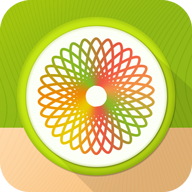# 万花尺画图软件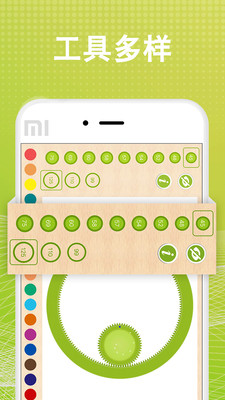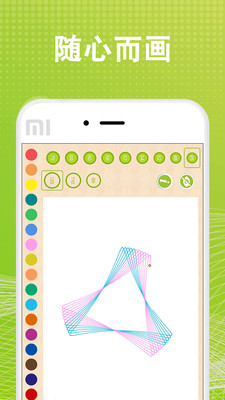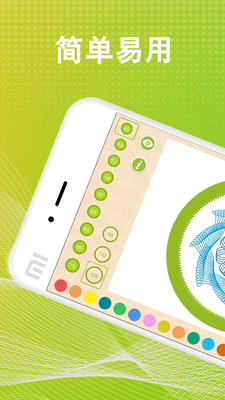### 官方简介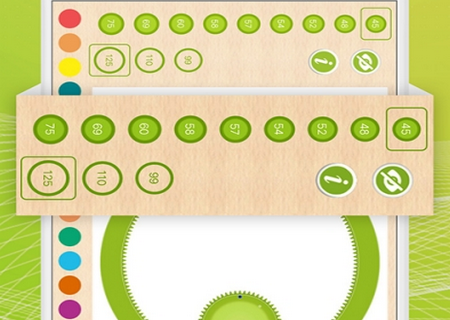### 相关新闻资讯

1、首先我们需要导入画图和数据计算的相关库。

import numpy as np

from numpy import sin, cos

from matplotlib import pyplot as plt

from matplotlib.font_manager import FontProperties

2、为了在图片上显示中文，需要先加载Alibaba-PuHuiTi-Medium字体。

wget http://tianchi-media.oss-cn-beijing.aliyuncs.com/DSW/Python/miniproject/01_draw_moon_cake/Alibaba-PuHuiTi-Medium.ttf

3、这里是核心方法，根据数学中内旋轮线（hypotrochoid）参数方程，利用lambd函数生成坐标点：

x = lambda d,r,R,theta: (R-r)*np.cos(theta) + d*np.cos(((R-r)/r)*theta)

y = lambda d,r,R,theta: (R-r)*np.sin(theta) - d*np.sin(((R-r)/r)*theta)

4、接下来我们要画摆线，摆线是月饼边缘的花纹，首先添加相关参数，参数说明见注释： 这个参数是核心，理论上通过玄学调参可以生成无数种可能。可以尝试发挥。例如用for循环遍历 生成渐变动画～

# 转数-内圆旋转次数

revs = 30

# 迭代次数， 即沿绘制路径获取的点。

Niter = 9999

thetas = np.linspace(0,revs*2*np.pi,num=Niter)

d = 2  #  距离

r = 11 # 小圆半径

R = 12 # 大圆半径

5、生成摆线花纹, 可通过color和linewidth参数调整颜色和线宽。

plt.plot(x(d, r, R, thetas), y(d, r, R, thetas), color='orange', linewidth = '4')

6、然后我们再画一个圆，这个圆是月饼的主体部分。

# 画个圆

length = 2.6

theta = np.linspace(0, 2 * np.pi, 100)

x = length * cos(theta)

y = length * sin(theta)

plt.plot(x, y, color='orange', linewidth = '5')

plt.axis('equal')

7.绘制文字，使用fontproperties参数可以使用上面下载的中文字体，因为代码不多，所以把剩余所有代码都写在这里：

import numpy as np

from numpy import sin, cos

from matplotlib import pyplot as plt

from matplotlib.font_manager import FontProperties

x = lambda d,r,R,theta: (R-r)*np.cos(theta) + d*np.cos(((R-r)/r)*theta)

y = lambda d,r,R,theta: (R-r)*np.sin(theta) - d*np.sin(((R-r)/r)*theta)

# 转数-内圆旋转次数

revs = 30

# 迭代次数， 即沿绘制路径获取的点。

Niter = 9999

thetas = np.linspace(0,revs*2*np.pi,num=Niter)

d = 2  #  距离

r = 11 # 小圆半径

R = 12 # 大圆半径

plt.plot(x(d, r, R, thetas), y(d, r, R, thetas), color='orange', linewidth = '4')

length = 2.6

# 画个圆

theta = np.linspace(0, 2 * np.pi, 100)

x = length * cos(theta)

y = length * sin(theta)

plt.plot(x, y, color='orange', linewidth = '5')

plt.axis('equal')

plt.axis('off') # 隐藏坐标系

font_set = FontProperties(fname=r"Alibaba-PuHuiTi-Medium.ttf", size=12)

plt.text(-1.2, -1, '中秋\n快乐', bbox=dict(boxstyle='circle', fc="w", ec='orange', linewidth=4), fontproperties=font_set, fontsize=40, color='orange') ##ec为线条颜色，color为字体颜色,可以自由替换

plt.text(-3, -4, 'Python画月饼，Spaceack与您千里共禅娟', fontproperties=font_set, fontsize=20, color='#aa4a30')

plt.show()